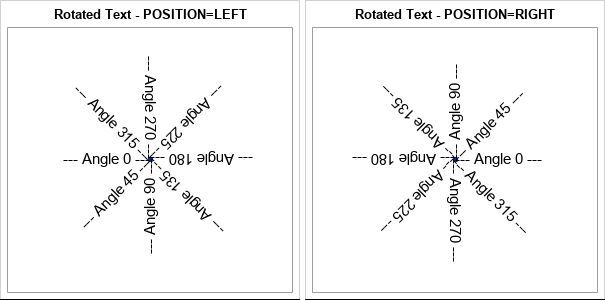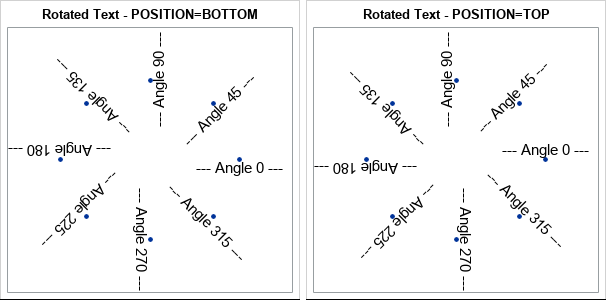The TEXT statement in PROC SGPLOT supports the ROTATE= option to rotate the specified text. It is worth knowing how the ROTATE= option interacts with the POSITION= option, which determines the anchor point at which the text is positioned. Briefly, the text is positioned FIRST, then the rotation occurs. The following list summarizes the interaction for common choices of the POSITION= option:

• POSITION=LEFT: The text is positioned to the left of the anchor point, then rotated around the anchor point. For example, if you rotate by 90 degrees, the text will be below the anchor point.
• POSITION=RIGHT: The text is positioned to the right of the anchor point, then rotated around the anchor point. For example, if you rotate by 90 degrees, the text will be above the anchor point.
• POSITION=BOTTOM: The text is positioned below the anchor point, then rotated around the anchor point. For example, if you rotate by 90 degrees, the text will be to the right of the anchor point.
• POSITION=TOP: The text is positioned above the anchor point, then rotated around the anchor point. For example, if you rotate by 90 degrees, the text will be to the left of the anchor point.

This summary is best illustrated by showing examples of rotated text. In the following example, the anchor point is always (0,0). The program uses either POSITION=LEFT or POSITION=RIGHT to positions a text string, which is then rotated through a series of angles.

```data TextRotate; retain x y 0; length Labl \$ 30; do Angle = 0 to 315 by 45; Labl = catx(' ', '---', 'Angle', Angle, '---'); output; end;   ods graphics / width=300px height=300px; %macro RotateTextPlot(position); title "Rotated Text - POSITION=&position"; proc sgplot data=TextRotate noautolegend; scatter x=x y=y / markerattrs=(symbol=CircleFilled); text x=x y=y text=labl / rotate=Angle position=&position textattrs=(size=12); yaxis display=none; run; %mend;   %RotateTextPlot(LEFT); %RotateTextPlot(RIGHT);```In a similar way, the following DATA step creates a series of anchor points on the unit circle. The program uses either POSITION=BOTTOM or POSITION=TOP to positions a text string, which is then rotated by an angle.

```data TextRotate; length Labl \$ 30; pi = constant('pi'); do Angle = 0 to 315 by 45; x = cos(Angle*pi/180); y = sin(Angle*pi/180); Labl = catx(' ', '---', 'Angle', Angle, '---'); output; end;   %RotateTextPlot(BOTTOM); %RotateTextPlot(TOP);```Be aware that this behavior is different from the way that text rotation works in SG annotation. To see how the anchor point (the value of the ANCHOR variable) interacts with the rotation angle (the ROTATE variable) in an SG annotation, see pp. 113–116 in Warren Kuhfeld's free e-book, Advanced ODS Graphics Examples, which inspired the graphs in this blog post. Briefly, if you anchor text on the left, then the text is positioned to the right, and vice versa.

ShareDistinguished Researcher in Computational Statistics

Rick Wicklin, PhD, is a distinguished researcher in computational statistics at SAS and is a principal developer of SAS/IML software. His areas of expertise include computational statistics, simulation, statistical graphics, and modern methods in statistical data analysis. Rick is author of the books Statistical Programming with SAS/IML Software and Simulating Data with SAS.

1.•